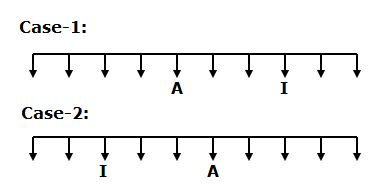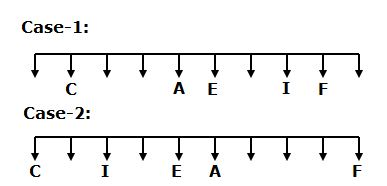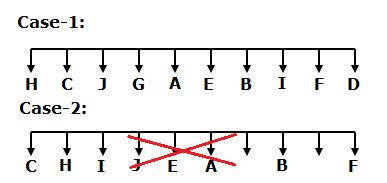# CWC/FCI Prelims 2019 Reasoning Ability Questions (Day-18)

Dear Readers, Exam Race for the Year 2019 has already started, To enrich your preparation here we are providing new series of Practice Questions on Reasoning Ability– Section for CWC/FCI Exam. Aspirants, practice these questions on a regular basis to improve your score in aptitude section. Start your effective preparation from the right beginning to get success in upcoming CWC/FCI Exam.

[WpProQuiz 5622]

Direction (1-5): Read the following information carefully and answer the questions given below.

Ten persons A, B, C, D, E, F, G, H, I and J are sitting in a straight row facing south but not necessary in the same order. Only two persons are sitting between A and I, who sits third from either ends of the rows. C sits fourth to the right of E. A is an immediate neighbour of E. As many sitting to the right of C is same as the persons sitting to the left of F. J sits second to the left of H. Only three persons are sitting between B and J. As many persons sitting between B and D is same as sitting between G and H.

1) Who among the following sits fourth to the left of A?

a) H

b) C

c) B

d) F

e) D

2) How many persons are sitting between D and E?

a) One

b) Two

c) Three

d) More than three

e) None

3) If A is related to B and I is related to D in a certain way. Then, G is related to which of the following?

a) J

b) C

c) E

d) F

e) H

4) Which of the following statements is true?

a) All are true

b) I is an immediate neighbour of B

c) H sits at one of the extreme ends

d) Only two persons are sitting to the right of J

e) G sits second to the right of E

5) Which of the following pairs sits at extreme ends of the rows?

a) H, C

b) D, H

c) H, A

d) D, B

e) None of these

Directions (6-10): In these questions relationship between different elements is shown in the statements. These statements are followed by conclusions. You have to find out which of the conclusions follow or not from the given statements. Give answer,

(a) If only conclusion I is true

(b) If only conclusion II is true

(c) If either conclusions I or II is true

(d) If neither conclusion I nor II is true

(e) If both conclusions I and II are true

6) Statement: G≤H<J=D≥A; B=N>D≥T≥S.

Conclusions:

I. H<B

II. S≤A

7) Statement: D=F>G≥H≤J; L≤K<M≥N=G.

Conclusions:

I. H<K

II. M≥D

8) Statement: Q<W>E≥R≤T; Y=U≤E<O≤P.

Conclusions:

I. P≥T

II. Y<W

9) Statement: L≥K≥J=H≤G; A>S<D≤H>M.

Conclusions:

I. K>M

II. L≥D

10) Statement: V>B=N≥M≤Z; Y<H≥B=W≥F.

Conclusions:

I. M≤F

II. F<V

Direction (1-5):• Only two persons are sitting between A and I, who sits second from either ends of the rows.• C sits fourth to the right of E. A is an immediate neighbour of E. As many sitting to the right of C is same as the persons sitting to the left of F.• J sits second to the left of H. Only three persons are sitting between B and J. As many persons sitting between B and D is same as sitting between G and H.
• So, Case-2 will be dropped.I. H<B –> H<J=D<N=B

II. S≤A –> A≤D≥T≥S

I. H<K –> H≤G=N≤M>K

II. M≥D –> M≥N=G<F=D

I. P≥T –> P≥O>E≥R≤T

II. Y<W –> Y=U≤E<W

I. K>M –> K≥J=H>M

II. L≥D –> L≥K≥J=H≥D